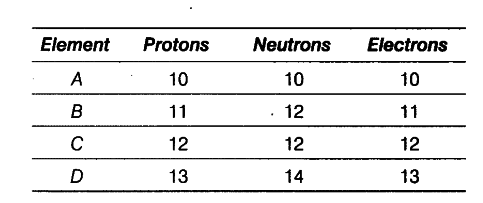# Write the electronic distribution of atoms of element A and D

The following data represents the distribution of electrons, protons and neutrons in atoms of four elements A, B, C, D.Solve the following questions.
(i) Write the electronic distribution of atoms of element A and D.
(ii) Element A is an inert gas. Why?
(iii) What is the valency of element C?

(i) Electronic distribution of element A and D.
K L M
A = 2,8
D = 2,8,3
(ii) The number of electrons in the outermost shell of element A is 8. The outermost shell of this element is complete and the element does not gain or lose electrons to complete its outermost shell. Hence, A is an inert gas.
(iii) Valency of element C (2, 8, 2) is 2.